Courses

# Chapter Notes - Production Function Commerce Notes | EduRev

## Commerce : Chapter Notes - Production Function Commerce Notes | EduRev

The document Chapter Notes - Production Function Commerce Notes | EduRev is a part of the Commerce Course Economics Class 12.
All you need of Commerce at this link: Commerce

MEANING :: It refers to functional relationship between the PHYSICAL INPUT used and PHYSICAL OUTPUT produced by firm. It is denoted as

 Qx = f (X1, X2, X3 ....) i.e output depends on various inputs (factor as well as non factors)

On assumption of input to be only labour and capital. Production function can be expressed as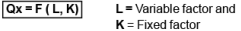It is a TECHNICAL RELATIONSHIP THAT TELLS MAXIMUM OUTPUT THAT CAN BE PRODUCED FROM GIVEN INPUTS,
Example 50 X = f (10 L, 2K) indicates that maximum of 50 units of commodity can be produced with 10 units of  labour and 2 units of capital.

SHORT RUN :: It refers to time period when a firm cannot vary all the input and thus amount of some inputs called “Fixed factors”  remains fixed.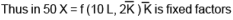LONG RUN :: It refer to time period when a firm can vary all the inputs used in production

 BASIS SHORT RUN LONG  RUN MEANING It refers to a period where output can be changed by changing only Variable factor It refers to a period where output can be changed by changing  all factors of production CLASSIFICATION There are Fixed and Variable factor in short period All factors are Variable factors in long run PRICE DETERMINATION Demand is more active in price determination as supply cannot be increased immediately with increase in demand Both Demand and supply are equally important in price determination as both can be increased

 The period is rather a functional concept which depends on production conditions. It varies from firm to firm and industry to industry . For example : a period of 10 years may be short period for a steel industry while a period of one year may be a long run period for a wheat producer.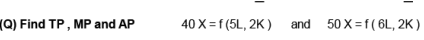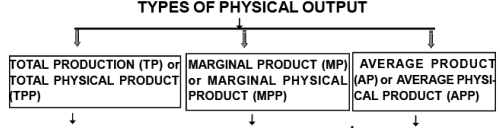It refers to total amount of goods produced in a gi ven period with given resources of variable inputs combined with fixed factors.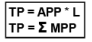EXAMPLE : A f armer by employing 1 unit of labour on land produces 5 quintal of It refers to change in totalproduction due to application of one more unit of variable factor other factor remaining constant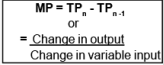Ex:  - If on same land father is joined by his son and thus 2 units of labour produces 7 quintal of wheat thenMP = 7-5 = 2 quintals It ref ers p er unit production of variable factor used in the process of production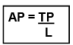Example ::  AP of father and son is  = 7/2 =  3.5 quintals

RELATION BETWEEN AP AND MP

(1) Both are derived from TP as MP = TPn - TPn -1  and    AP = TP / L

(2) AP is rising MP is above it

(3) MP cuts AP when AP is at maximum point

(4) AP is falling MP is below it

(5) MP can be zero and negative but AP cannot be.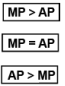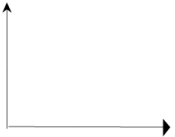THAT MP CAN FALL WHEN AP IS RISING :: MP reaches its maximum point earlier than AP as it is faster and thereafter starts falling from point “A” to “C” where as AP is rising from “B” to “C”. Thus it can be concluded that MP can fall when AP is rising

 SHAPE OF AP AND MP IS INVERSE U - SHAPE

WHY MP IS FASTER :: It must be noted that both AP and MP are derived from TP.

AP is calculated on the basis of ALL THE UNITS whereas
MP is based on ADDITIONAL UNIT ONLY.
So , it is the MP THAT PULLS THE AP UP OR DOWN

WHY MP CUT AP AT ITS MAXIMUM :: It happens because when AP rises , MP is more than AP. W hen AP falls , MP is less than AP. So it is only when AP is constant and at its  maximum point that MP is equal to AP. Therefore , MP cuts AP curve at its maximum point

WHY MP IS NEGATIVE :: MP is negative due to EXCESSIVE EMPLOYMENT or DISGUISED EMPLOYMENT (as in case of Agriculture sector or Public Sector Undertaking).
Excessive employment which means employment of more workers than required reduces overall efficiency of the worker and makes MP negative

RELATIONSHIP BETWEEN MP AND TP

(1) TP = Σ MP = MP1 + MP2  + MP3 + ................ + MPn.

(2) TP is INCREASING AT INCREASING RATE WHEN MP IS POSITIVE AND INCREASING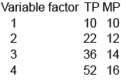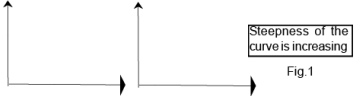(3) TP is INCREASING AT CONSTANT RATE WHEN MP IS POSITIVE AND CONSTANT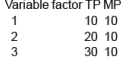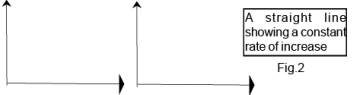(4) TP INCREASES AT DIMINISHING RATE MP IS POSITIVE BUT DECREASES

(5) TP is MAXIMUM WHEN MP IS ZERO (i.e MP touches X - axis)

(6) TP FALLS WHEN MP IS NEGATIVE (i.e below X - axis)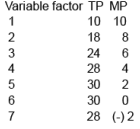Steepness of the curve is decreasing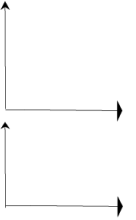MEANING :: It explain the BEHAVIOUR OF OUTPUT IN SHORT PERIOD when input of one factor is  increased while all other factor remains constant. In other words it TELLS CHANGES IN OUTPUT WHEN THERE IS CHANGE IN FACTOR RATIO as more and more of variable factor is employed with same fixed factors in short period.

(1) INCR EASING RETUR N TO FACTOR :: It re fers to situ ation when given percentage INCREASE IN VARIABLE FACTOR only causes proportionate MORE increase in output

In other words MARGINAL PRODUCT of the variable factor must be INCREASING and total output tends to increase at the increasing rate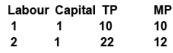(1) FULLER UTILISATION OF FIXED FACTORS :: When in short period more and more of variable factor is employed with same fixed factors (like machinery), the underutilised fixed  factors are better and fully utilised. This fuller utilisation increases efficiency of fixed factors and thus MP tends to increase
EXAMPLE :: a Small plant is manufacturing cloth. Five workers are required to get maximum output out of this plant. If this factory hires one or two workers the production would be inefficient.
These difficulties would disappear as more workers are added. The plant would be more efficiently utilized.

(2) INCREASE IN EFFICIENCY :: Several economists like Adam Smith, Marshall, and Mrs. Joan Robinson were of the opinion due to increase in the units of variable factor the possibility of division of labour and specialization increases. Thus good deal of saving would be possible with regard to training, equipment and time etc. and law of increasing returns would apply.

(3) BETTER COORDINATION BETWEEN FACTORS :: There is improved degree of coordination between variable and fixed factors which increases productivity and hence total output

(4) INDIVISIBILITY OF FIXED FACTOR :: It means to produced goods upto certain limit some fixed factor is must.
Example  :: An economic teacher is fully competent to teach 30 students with ease and efficiency. Now if number of student falls to 15, the teacher cannot be reduced partly

(2) CONSTANT RETURN TO FACTOR :: It refers to situation when given percentage INCREASE IN VARIABLE FACTOR only cause proportionate SAME increase in output

In other words MARGINAL PRODUCT of the variable factor must be CONSTANT andtotal output tends to increase at the constant rate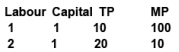CAUSES OF CONSTANT RETURNS(i)  Optimum Utilisation of the Fixed Factor(ii) Ideal Factor Ratio(iii) Most Efficient Utilisation of the Variable Factor

(3) DIMINSHING RETURN TO A FACTOR OR THE LAW OF DIMINISHING RETURNS :: Diminishing returns to a factor or law of Diminishing Returns refers to the situation when due to given percentage INCREASE IN VARIABLE INPUT only causes proportionally LESS increase in output.

⇒ In other words MARGINAL PRODUCT of the variable factor must be DECREASING, becomes zero and finally negative with every employment of variable factor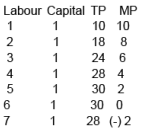This was initially called the LAW OF DIMINISHING MARGINAL RETURN BY MARSHAL as he applied this law to agriculture only but Joan Robinson, Stigler and other modern economist called it the LAW OF VARIABLE PROPORTION  WHICH COULDBE APPLIED IN ALL SECTORS of the economy and considered it to be universal law.

(1) FIXITY OF FACTOR ::  It refers to over-utilisation of fixed factors, beyond a certain level employment of more variable factor may lead to breakdown of fixed factor and thus stoppage in production occurs which results in increase in cost  and diminishing returns sets in.

(2) IMPERFECT FACTOR SUBSTITUTE :: Acc. to Mrs John Robinson since labour cannot be substituted for capital infinitely and more labour to same capital will result in decreasing return sooner or later

(3) POOR COORDINATION :: More Variable factor with Fixed factor distort the coordination and creates managerial problem. This results in declining TP

(4) BEYOND OPTIMUM PRODUCTION : After the optimum use of a fixed factor if it is combined with increasing units of variable factor, then the marginal return of such variable factor begins to diminish. It is so becuase after the optimum use, the ratio of fixed and variable factors becomes defective

For instance, a machine is a fixed  factor of production.It is put to optimum use when 4 labourers are employed onit. If fifth labourer is employed on it, then production will increase very little, because marginal product of this labourer will diminish.

LAW OF VARIABLE PROPORTION (LOVP)

MEANING :: This law states that as proportion of variable factor is changed
→ TPP increases at an increasing rate in the beginning
→ then increases at a diminishing rate and
→ after a certain point , it starts falling.

In other words when  variable factor is increased TOTAL PRODUCT CHANGES AT DIFFERENT RATES, THIS TENDENCY IS CALLED LOVP.

 It is also known as “LAW OF RETURN”or  “ LAW OF RETURN TO FACTOR ”

 SPECIAL POINT :: LOVP is an extension of Law of diminishing returns as it considers phases of rising as well as falling MP

ASSUMPTIONS ::
(1) One of the factors is variable while all other factors are fixed
(2) All units of the variable factor are homogenous or equally efficient.
(3) There is no change in the technique of production.
(4) Factors of production can be used in different propotion. For example 2 hectares of land with 1 labour ; or 2 hectares of land with 4 labourers
(5) Short period operates
(6) Adequate or standard doses of variable factor are applied.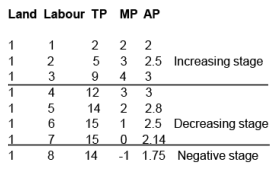(Note that TP is-  convex in phase 1-  concave in phase 2 and- downward sloping in phase 3.This is how we can identify the three phases.)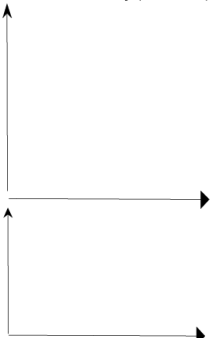STAGES TOTAL PRODUCT MARGINAL PRODUCT Increasing Return It increases from point “O” to point “E” at  increasing rate It increases upto Maximum point “G” Diminishing Return Increase at diminishing rate and reaches its maximum point “F” It decrease to point “H”and becomes zero (touches X-axi) Negative Return TP starts falling It becomes negative

STAGE OF OPERATION

STAGE 1st ::  The firm is moving towards optimum combination of factors as TP is increasing at an increasing rate and there is scope of more profit , so a firm will continue its production by employing additional variable factor and will increase its profitability.

STAGE IIIRD :: This stage results in less profit due to
(a) additional cost incurred by employing more variable factor
(b) Lesser revenue generated due to fall in output
Thus Stages Ist and IIIrd are therefore called stages of economic absurdity

STAGE IIND :: A firm has reaped the benefit of increasing return and is moving towards negative return, therefore it will choose its volume of production in this stage depending upon its production policy and this stage is referred to as LAW OF DIMINISHING MARGINAL RETURN as this states that increasing the employment of an input, with other input fixed, eventually a point will be reached after which the RESULTING ADDITIONAL OUTPUT WILL STARTS FALLING.

POSTPONEMENT OF THE LAW

(i) The LOVP becomes inoperative when IMPROVED TECHNOLOGY is introduced causing increase in productivity and fall in cost

(ii) The operation of the law may be postponed also when the FACTORS OF PRODUCTION ARE PERFECT SUBSTITUTE OF EACH OTHER. In such a situation the limitation of the fixity of factor does not exist

Offer running on EduRev: Apply code STAYHOME200 to get INR 200 off on our premium plan EduRev Infinity!

## Economics Class 12

200 videos|214 docs|62 tests

,

,

,

,

,

,

,

,

,

,

,

,

,

,

,

,

,

,

,

,

,

;